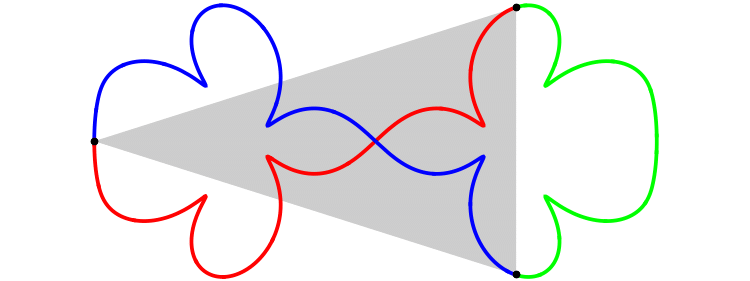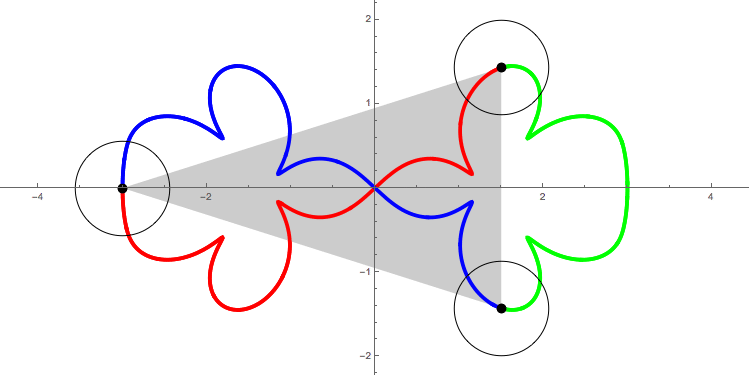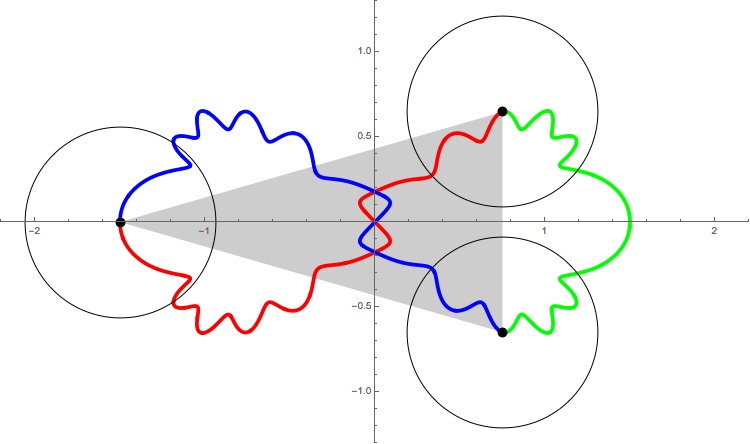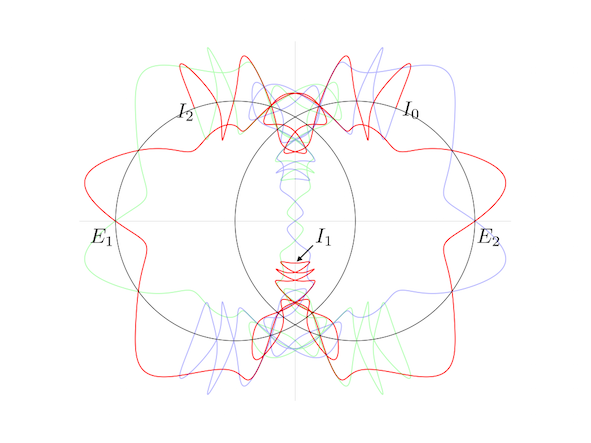# Animation for some figure-eight solutions under Lennard-Jones potential

See also Hiroshi Fukuda's site Three Body Problem'' http://kilin.clas.kitasato-u.ac.jp/3body/Hiroshi Fukuda found many figure-eight solutions under Lennard-Jones potentail U=1/r^{12}-1/r^6.
The preprint
 H. Fukuda, T. Fujiwara and H. Ozaki, Figure-eight choreographies of the equal mass three-body problem with Lennard-Jones-type potentials, http://arxiv.org/abs/1606.08760
You can see many series for figure-eights in this paper.

The existence of figure-eight solutions under Lennard-Jones potential was predicted by L. Sbano in his talk at SPT 2004 held at Cala Gonone, Italy. He predicted that there are at least two solutions for sufficiently large period''.
 L. Sbano, Symmetric solutions in molecular potentials, Proceedings of the international conference SPT 2004, World Scientific, 2005
 L. Sbano and J. Southall, Periodic solutions of the N-body problem with Lennard-Jones type potentials, Warwick preprint, June 2007
 L. Sbano and J. Southall, Periodic solutions of the N-body problem with Lennard-Jones type potentials, Dynamical Systems Vol. 25 #1, 2010

The followings are animations for some figure-eights that are found by Hiroshi Fukuda.

• Animation 1: (x_0, y_0, E)=(1.5, 1.427721000, 0.000122434)
T=341.278The three black points represent the position of q_k, k=0, 1, 2. As you see in the movies, the three bodies bounce as if they were balls with radious r_0=2^{1/6}/2=1.122... that are represented by circles. Two bodies feel repulsive force, when the distance between them is smaller than 2 r_0=2^{1/6}. Otherwise, they feel attractive force. See the following figures for U(r), repulsive case, and attractive case.• Animation 2: (x_0, y_0, E)=(0.75, 0.64808093, -0.14752617)
T=44.485• Animation 3: (x_0, y_0, E)=( 0.473207906706962783102256117707, 1.01061071815628640266937160262, -0.2)
T=111.029The following figure shows the orbit of the figure-eight of animation 3 in the shape plane'', q_3(t)/(q_2(t)-q_1(t)).

If you need more precise picture, follow this link 20160703LennardJonesFig3Shape3.pdfThe X-axis is the equator of the shape sphere''. Any points on the equator represents colinear configuration. The Y-axis and two circles are the lines of longitude of the shape sphere'', whose mutual angle is equal to 2\pi/6. Any point on these five longitude lines represents isosceles configuration.

The red curve represents the interval of time [0, T/3]. And green and blue one represent [T/3, 2T/3] and [2T/3, T] respectively.

See the red curve.
At t=0, the motion starts at the isosceles configuration I_0. At t=T/12, it passes through the Euler point E_1. Then at 2T/12, I_1. Namely, in short expression, I_0(t=0) --> E_1(T/12) -->I_1(2T/12) --> E_2(3T/12) --> I_2(4T/12=T/3)

In the interval of time [0, T/3], the motion passes through just two Euler points E_1 and E_2. For isosceles configurations, although the motion passes through many isosceles configurations, only pathes at I_0, I_1 and I_2 are parpendiculer to the longitude line. This is the requirement for the figure-eight solution. So, this solution has a qualification to be called one of the figure-eight solution''.